Customer/Vendor Loan

## Customer / Vendor Loan

### This Application helps to track record of customer loan and it's data.

Basic Features
• This application is allow you to manage customer and vendor(bank) Loans
Notes :
• For This application User need to install numpy python library
• Install it from sudo pip install numpy or sudo apt install python-numpy or sudo apt install python3-pip(For python3)
Look Below Screenshots: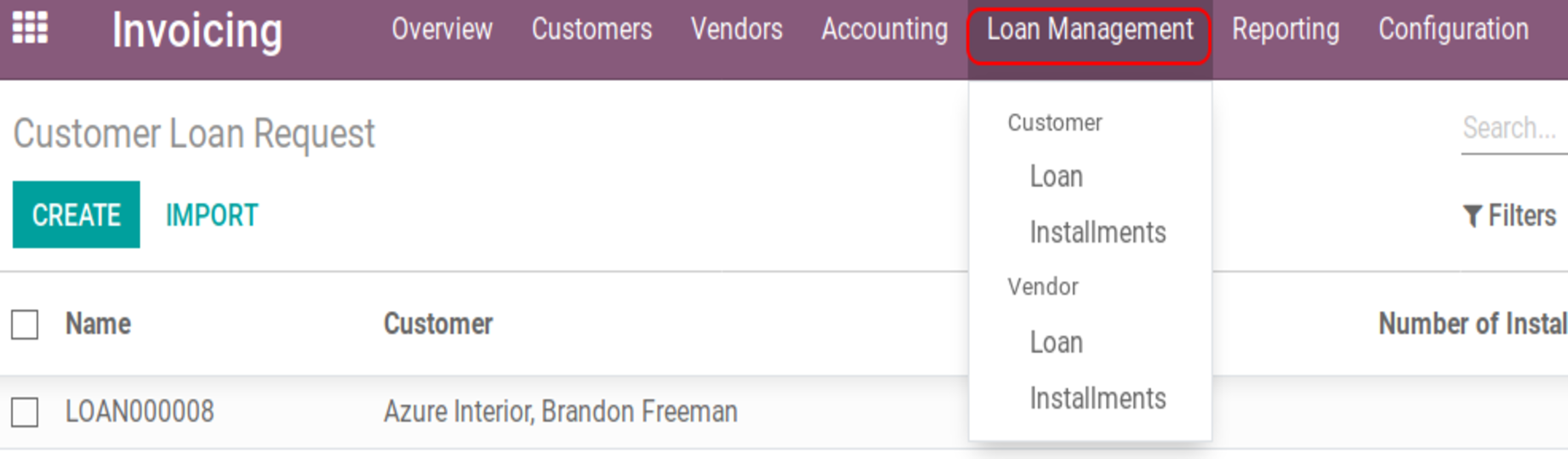• Loan Management will display under Invoicing Menu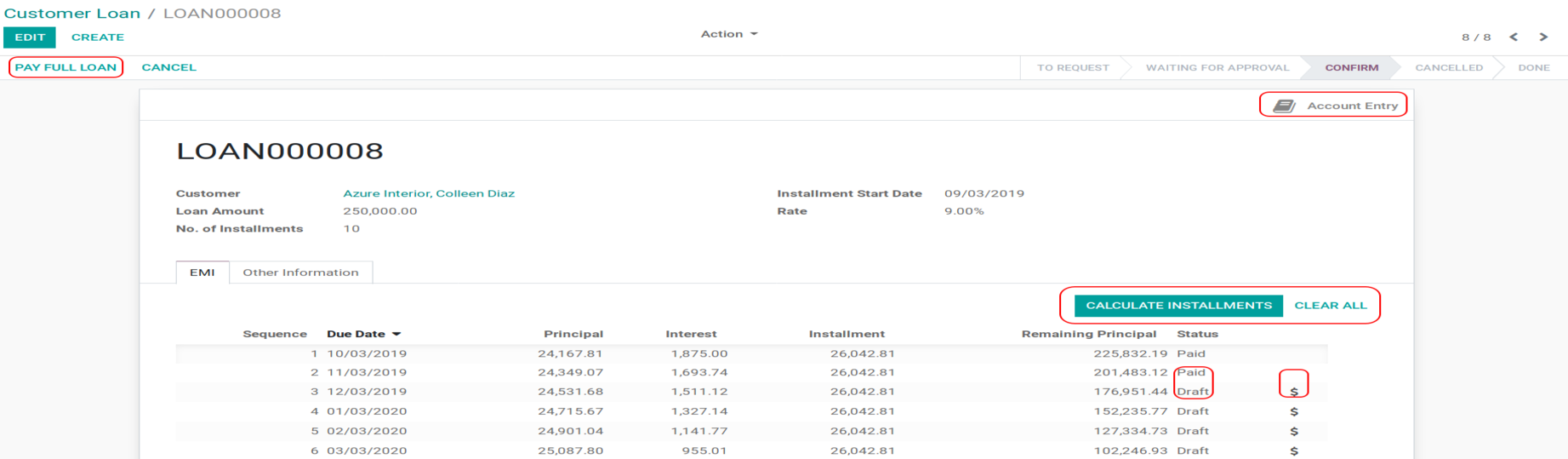Above Image Is customer loan.Above Image Is for accounting configuration.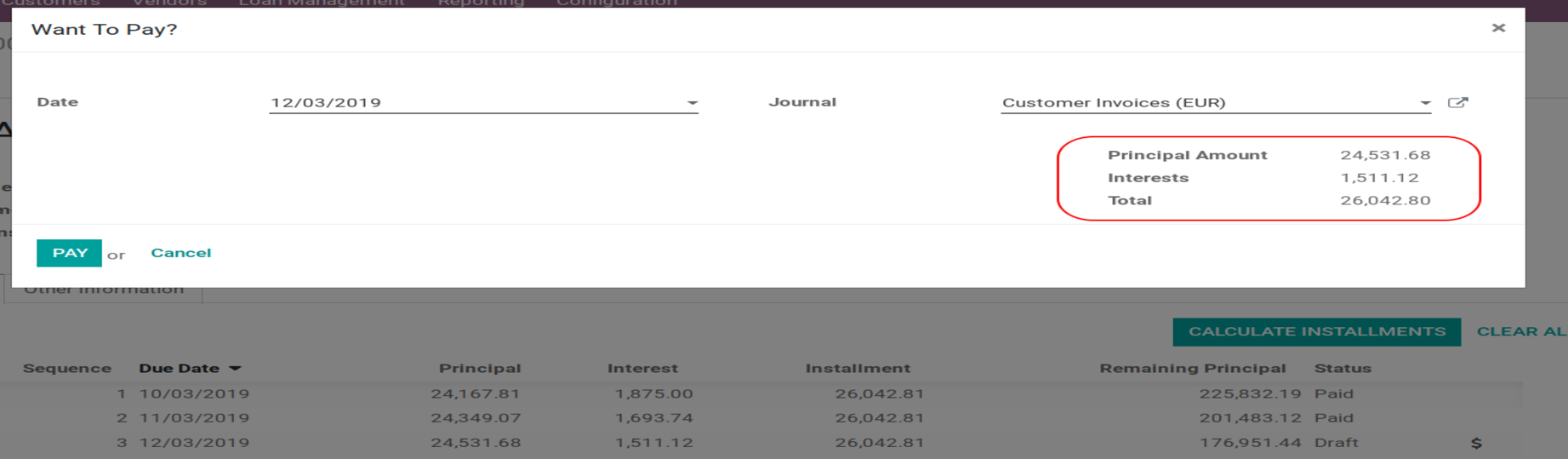Above Image Is display if user want to pay any installment and related amount will display in that popup.Above Image Is for user want to pay full loan in one payment. Then pending interest is not added in that popup. If company not want to add interest on that transction or want to add low interest.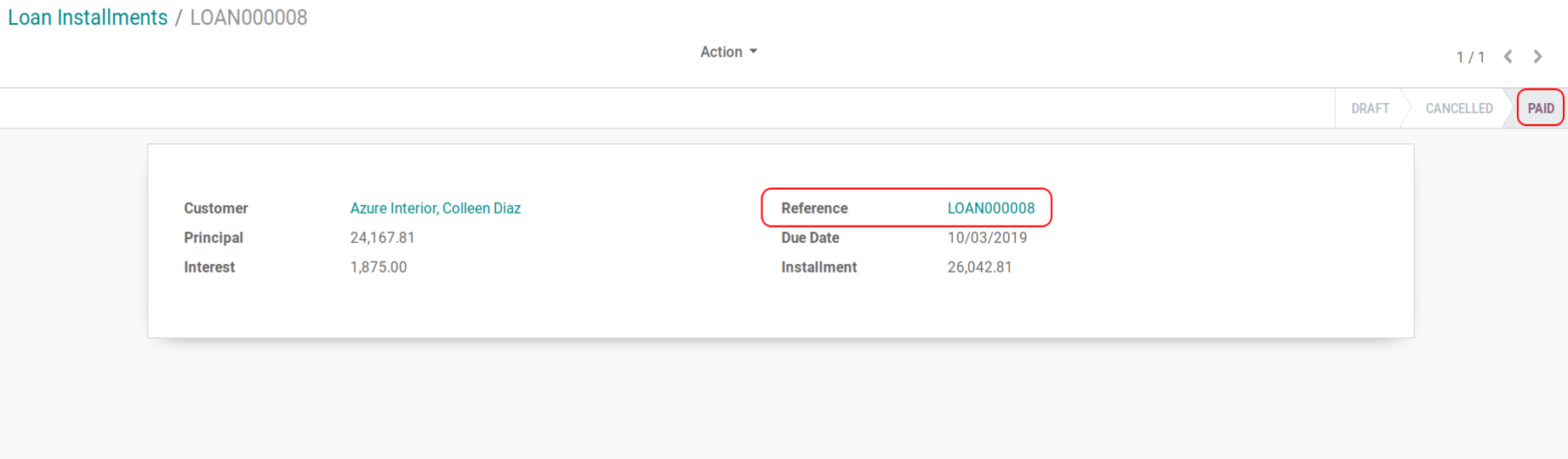Above Image Is display installment view.Above Image Is for advance configuration add configurable interval in EMI(Installment Date). Invoicing --> Configuration --> Loan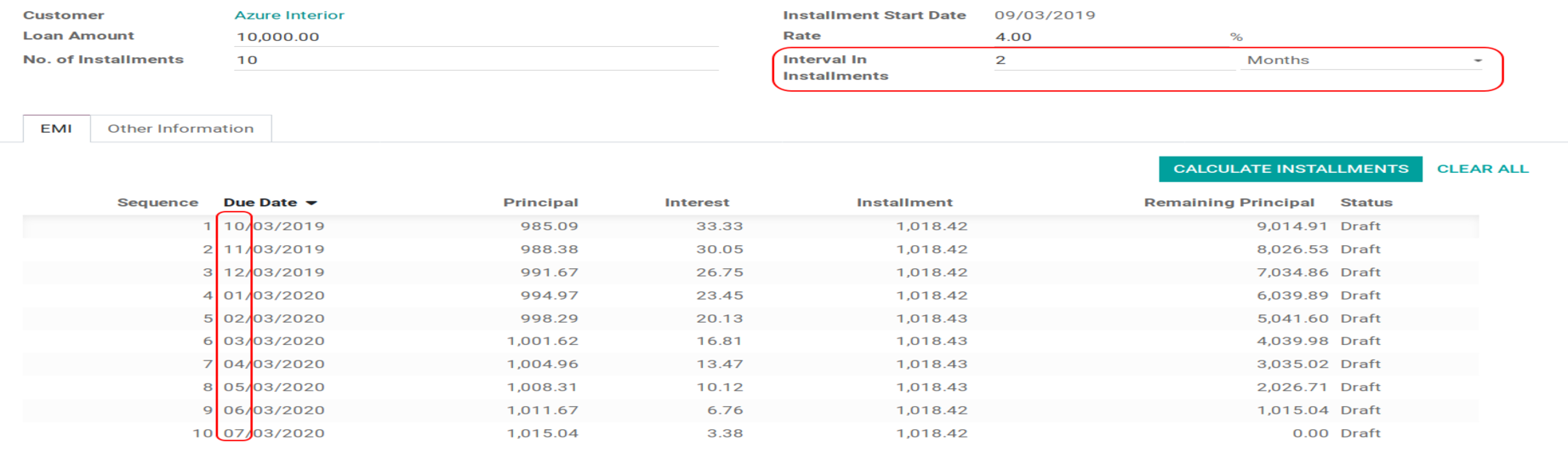Above Image Is for if user want to add 2 months interval in installment. Or User want to change loan to calculate loan based on date or in year.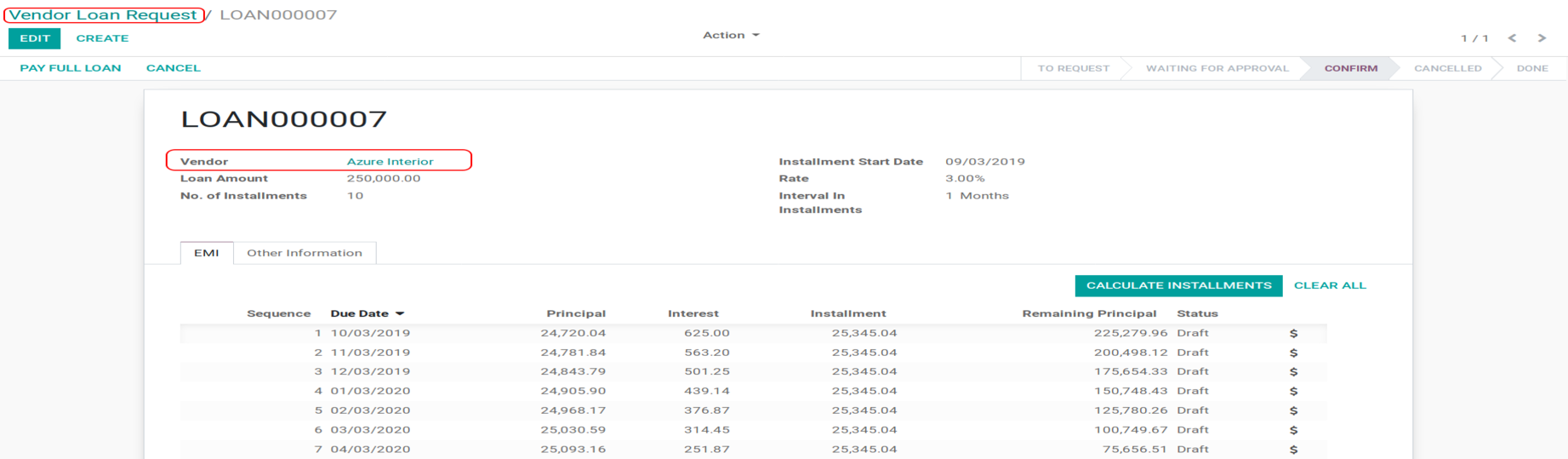Above Image Is for Vendor Loan or Bank loan tracking.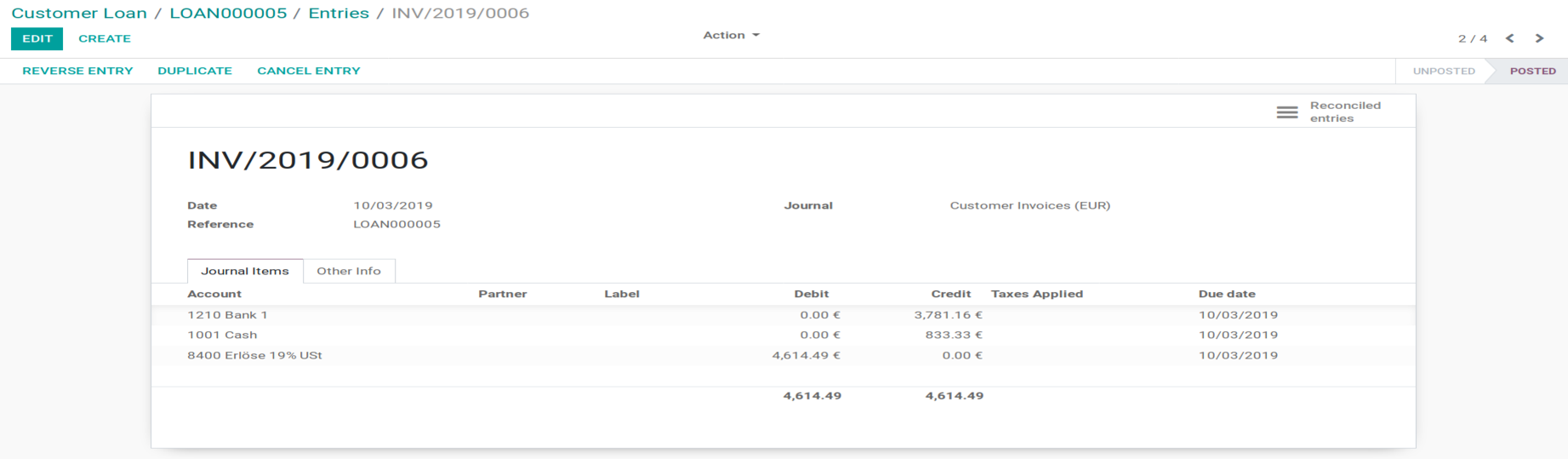Accounting Entry of installment.
Example with equation: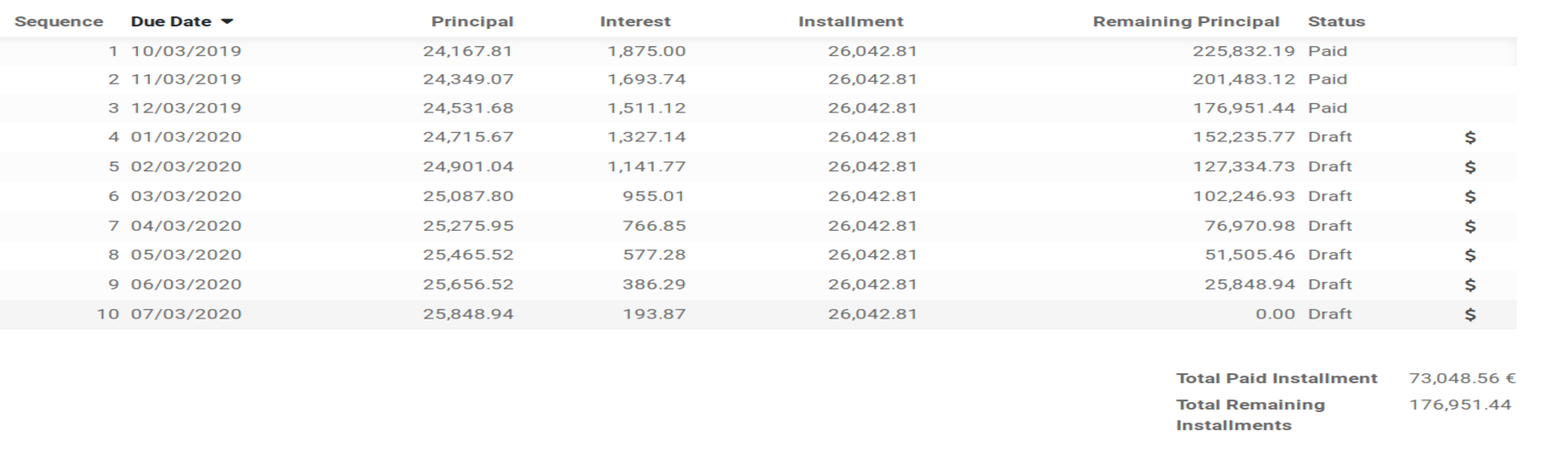I = PRN/100

E is EMI

I is for Interest

P is Principal Loan Amount

r is rate of interest calculated on monthly basis. (i.e., r = Rate of Annual interest/12/100. If rate of interest is 9% per annum, then r = 9/12/100=0.0075) n is loan term / tenure / duration in number of months

Loan for 10 months

N = 1/12 for monthly intrest P = 2,50,000 R = 9%

Equation:

I = 250000*9 / 100*12

I1 = 1875

Above screen is show calculation by month :

If rate is 9 %

Per month rate of interest is 0.0075

1st month principal amount is 2,50,000

Payment amount

EMI = P*r*(1+r)^n /((1+r)^n-1*(1 /100)

First Installment Calculation

250000*0.0075*(1 + 0.0075)^10 / ((1 + 0.0075)^10-1) = 26042.81

Installment amount = 4612.49 Interest amount = 1875.00 Principal amount = 3781.16

So next month Remaining Amount is 2,50,000 - 24167.81 = 225,832.19

Next month Interest amount = 225,832.19 * 9 * 1 / 12/ 100 = 1693.74

Second Installment Calculation

225,832.19*0.0075*(1 +0.0075)^9 / ((1 + 0.0075)^9 - 1) = 26042.81

## Complimentary Support

You will get 90 days free support for any doubt, queries, and bug fixing (excluding data recovery) or any type of issue related to this module.